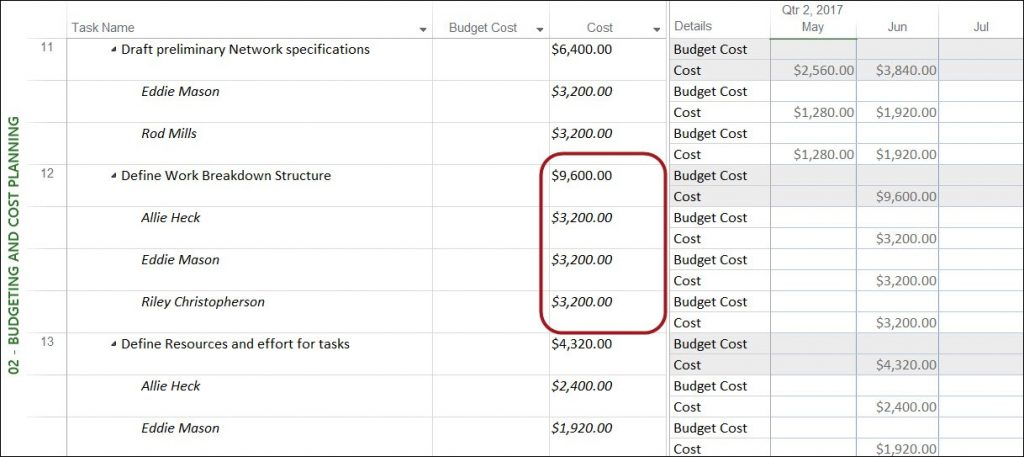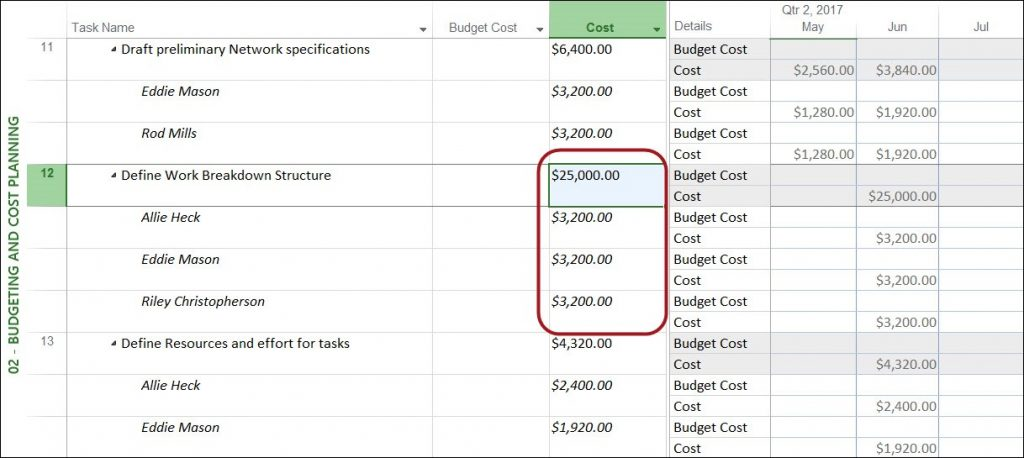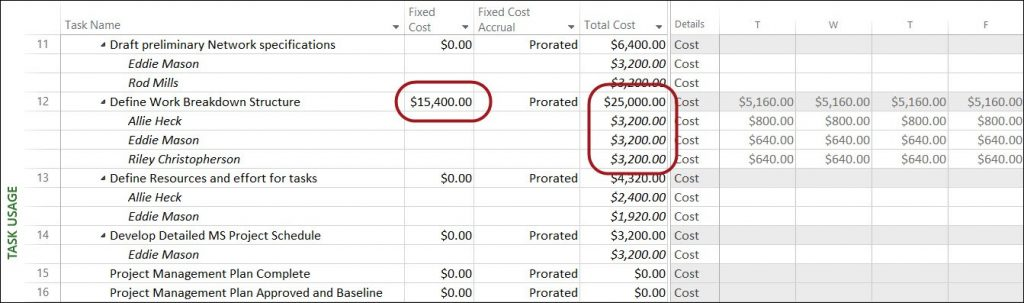Manually Enter Task Costs – How Is This Even Possible? | Sensei Project Solutions

Sensei Blog & News

The blog about Microsoft productivity, project and portfolio management and business insights solutions.

By Dale Howard, Director of Education for Sensei Project Solutions.

I learned something new last week about Microsoft Project. This new knowledge is an extension of how Microsoft Project calculates the Cost value for each task. As many of us already know, the software calculates the Cost value for each task as the sum of the Cost values for each resource assigned to the task. For example, in the following figure, notice that Cost value for the Define Work Breakdown Structure (\$9,600) is simply the total of the Cost values for the three resources assigned to this task (\$3,200 for each of the three resources). This is the behavior that we expect to see in Microsoft Project.Figure 1 – Task Cost is the Sum of Assignment Costs

Last week one of our clients ran into an interesting situation with the task Cost field, when they discovered that one of their project managers had manually typed the Cost value for a task. What the client saw in the project is very similar to the project shown in Figure 2. The client had a custom view applied, which in this case was the 02 – Budgeting and Cost Planning view. Notice that this view shows the Budget Cost and Cost columns for each task. Notice that the Cost value for the Define Work Breakdown Structure task (\$25,000) is not the sum of the Cost values for the three assigned resources (it should be \$9,600).Figure 2 – Manually Entered Task Cost Value

As you might surmise, when the client saw this in the project, they were very concerned.  They reached out to our Team to find out how it is possible for a project manager to manually type a Cost value for an individual task. They questioned whether this was a bug (aka a “feature”) in the software, or somehow by design. I love “brain teasers” like this, so I gladly accepted the challenge to solve this problem.

The first thing I did was apply the default Task Usage view and then apply the default Cost table. With those two simple steps, I was able to quickly determine what happens when a project manager manually types a task Cost value. In Figure 3, notice that when I manually typed \$25,000 in the Cost column, Microsoft Project calculated a Fixed Cost value of \$15,400 for this task.Figure 3 – Calculated Fixed Cost Value

Total Cost values for each resource assigned to the task, plus any Fixed Cost amount associated with the task. When I manually typed the \$25,000 value in the Total Cost column for the task, Microsoft Project assumed that there is an additional cost for this task, and the software entered this additional cost in the Fixed Cost column! The \$15,400 value in the Fixed Cost column is the difference between the value I manually entered in the Total Cost column (\$25,000) and the calculated total of the Total Cost values for the three resources assigned (\$9,600).

So, I think you would agree that this behavior is definitely not a bug, and is certainly by design.  Keep in mind that there is no way to prevent a project manager from manually typing a task Cost value, so keep your eyes on the Fixed Cost column for any projects you audit.